# Differentiability - JEE Main Previous Year Question with Solutions

JEE Main Previous Year Question of Math with Solutions are available at eSaral. Practicing JEE Main Previous Year Papers Questions of mathematics will help the JEE aspirants in realizing the question pattern as well as help in analyzing weak & strong areas. eSaral helps the students in clearing and understanding each topic in a better way. eSaral is providing complete chapter-wise notes of Class 11th and 12th both for all subjects. Besides this, eSaral also offers NCERT Solutions, Previous year questions for JEE Main and Advance, Practice questions, Test Series for JEE Main, JEE Advanced and NEET, Important questions of Physics, Chemistry, Math, and Biology and many more. Download eSaral app for free study material and video tutorials.
Q. Let $\mathrm{f}(\mathrm{x})=\mathrm{x}|\mathrm{x}|$ and $\mathrm{g}(\mathrm{x})=\sin \mathrm{x}$ Statement–1 : gof is differentiable at x = 0 and its derivative is continuous at that point. Statement–2 : gof is twice differentiable at x = 0. (1) Statement–1 is true, Statement–2 is false. (2) Statement–1 is false, Statement–2 is true. (3) Statement–1 is true, Statement–2 is true; Statement–2 is a correct explanation for Statement– 1. (4) Statement–1 is true, Statement–2 is true ; Statement–2 is not a correct explanation for statement– 1. [AIEEE-2009]
Ans. (1) $F(x)=x|x| \quad g(x)=\sin x$ $\operatorname{gof}=\sin x|x|=\left[\begin{array}{cc}{2 x \cos x^{2}} & {x>0} \\ {-2 x \cos x^{2}} & {x<0}\end{array}\right.$ $(\mathrm{gof})^{\prime \prime}=\left[\begin{array}{cc}{2 \cos \mathrm{x}^{2}-4 \mathrm{x}^{2} \sin \mathrm{x}^{2}} & {\mathrm{x}>0} \\ {-2 \cos \mathrm{x}^{2}+4 \mathrm{x}^{2} \sin \mathrm{x}^{2}} & {\mathrm{x}<0}\end{array}\right.$ $\Rightarrow$ First derivative exist but second derivative doesn't exist.
Q. If function $\mathrm{f}(\mathrm{x})$ is differentiable at $\mathrm{x}=\mathrm{a}$ then $\lim _{x \rightarrow a} \frac{\mathrm{x}^{2} \mathrm{f}(\mathrm{a})-\mathrm{a}^{2} \mathrm{f}(\mathrm{x})}{\mathrm{x}-\mathrm{a}}$ (1) $2 \mathrm{a} \mathrm{f}(\mathrm{a})+\mathrm{a}^{2} \mathrm{f}^{\prime}(\mathrm{a})$ (2) $-a^{2} f^{\prime}(a)$ (3) af $(a)-a^{2} f^{\prime}(a)$ (4) $2 \mathrm{af}(\mathrm{a})-\mathrm{a}^{2} \mathrm{f}^{\prime}(\mathrm{a})$ [AIEEE-2011]
Ans. (4)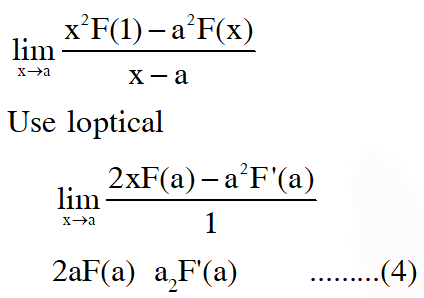Q. Consider the function, $f(x)=|x-2|+|x-5|, x \in R .$ Statement–1 : f'(4) = 0. Statement–2 : f is continuous in [2, 5], differentiable in (2, 5) and f(2) = f(5). [AIEEE 2012] (1) Statement–1 is true, Statement–2 is false. (2) Statement–1 is false, Statement–2 is true. (3) Statement–1 is true, Statement–2 is true ; Statement–2 is a correct explanation for Statement1. (4) Statement–1 is true, Statement–2 is true ; Statement–2 is not a correct explanation for Statement1. [AIEEE 2012]
Ans. (4)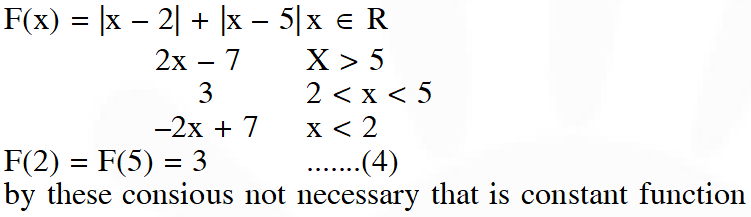Q. Let $\mathrm{f}(\mathrm{x})=\mathrm{x}|\mathrm{x}|, \mathrm{g}(\mathrm{x})=\sin \mathrm{x}$ and $\mathrm{h}(\mathrm{x})=(\mathrm{gof})(\mathrm{x}) .$ Then (1) h(x) is differentiable at x = 0 (2) h(x) is continuous at x = 0 but is not differentiable at x = 0 (3) h(x) is differentiable at x = 0 but h(x) is not continuous at x = 0 (4) h(x) is not differentiable at x = 0 [On-line 2014]
Ans. (2)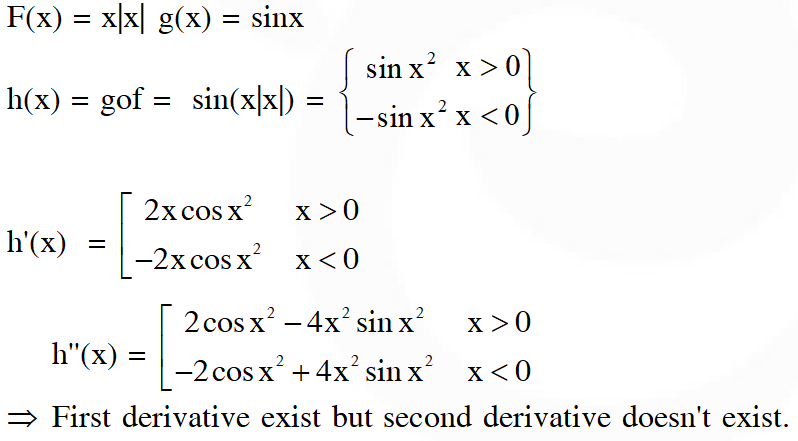Q. Let $\mathrm{f}, \mathrm{g}: \mathrm{R} \rightarrow \mathrm{R}$ be two functions defined byf(x) $=\left\{\begin{array}{ll}{\mathrm{x} \sin \left(\frac{1}{\mathrm{x}}\right), \mathrm{x} \neq 0} & {, \text { and } \mathrm{g}(\mathrm{x})=\mathrm{xf}(\mathrm{x}):-} \\ {0} & {, \mathrm{x}=0}\end{array}\right.$ Statement I : f is a continuous function at x = 0. Statement II : g is a differentiable function at x = 0. (1) Statement I is false and statement II is true (2) Statement I is true and statement II is false (3) Both statement I and II are true (4) Both statements I and II are false [on-line 2014]
Ans. (3)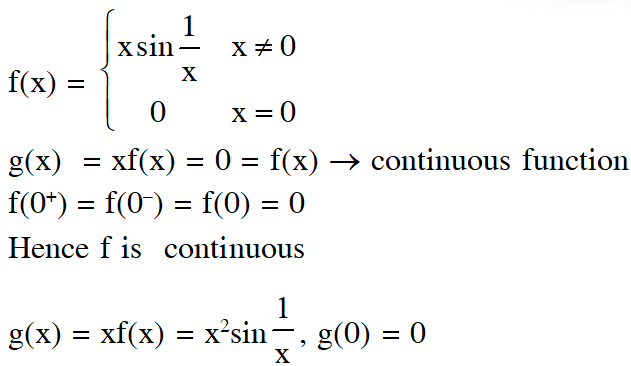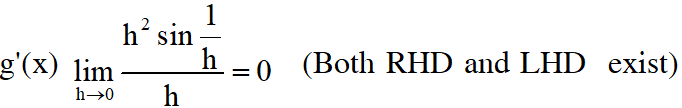Q. Let $\mathrm{f}: \mathrm{R} \rightarrow \mathrm{R}$ be a function such that $|\mathrm{f}(\mathrm{x})| \leq \mathrm{x}^{2},$ for all $\mathrm{x} \in \mathrm{R} .$ Then, at $\mathrm{x}=0, \mathrm{f}$ is: (1) Neither continuous nor differentiable (2) differentiable but not continuous (3) continuous as well as differentiable (4) continuous but not differentiable [on-line 2014]
Ans. (3) $|\mathrm{f}(\mathrm{x})| \leq \mathrm{x}^{2}$ $-\mathrm{x}^{2} \leq \mathrm{f}(\mathrm{x}) \leq \mathrm{x}^{2} \therefore$ at $\mathrm{x}=0 \mathrm{f}(\mathrm{x})$ is continuons as well as differentiable
Q. For $\mathrm{x} \in \mathrm{R}, \mathrm{f}(\mathrm{x})=|\log 2-\sin \mathrm{x}|$ and $\mathrm{g}(\mathrm{x})=\mathrm{f}(\mathrm{f}(\mathrm{x})),$ then : (1) $\mathrm{g}$ is differentiable at $\mathrm{x}=0$ and $\mathrm{g}^{\prime}(0)=-\sin (\log 2)$ (2) $\mathrm{g}$ is not differentiable at $\mathrm{x}=0$ (3) $\mathrm{g}^{\prime}(0)=\cos (\log 2)$ (4) $\mathrm{g}^{\prime}(0)=-\cos (\log 2)$ [JEE Mains 2016]
Ans. (3)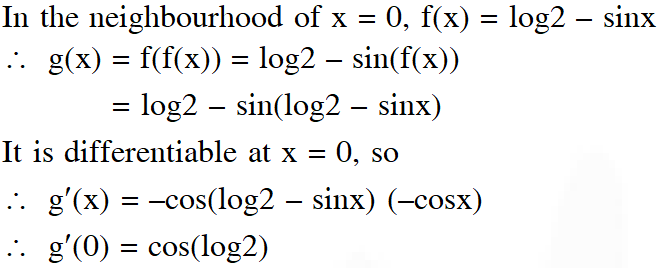Q. Let $\mathrm{S}=\left\{\mathrm{t} \in \mathrm{R}: \mathrm{f}(\mathrm{x})=|\mathrm{x}-\pi| \cdot\left(\mathrm{e}^{|\mathrm{x}|}-1\right)\right.$ $\sin |x|$ is not differentiable at t}. Then the set S is equal to: (1) {0}           (2) $\{\pi\}$           (3) $\{0, \pi\}$              (4) $\phi$ (an empty set) [JEE Mains 2018]
Ans. (4)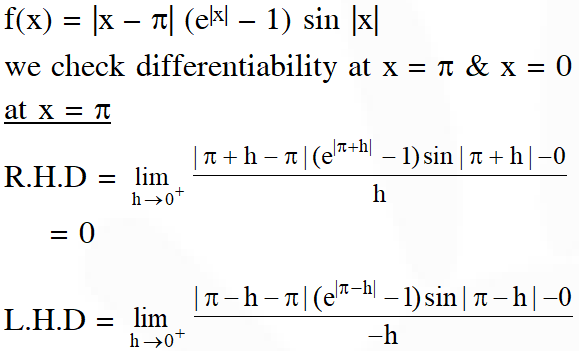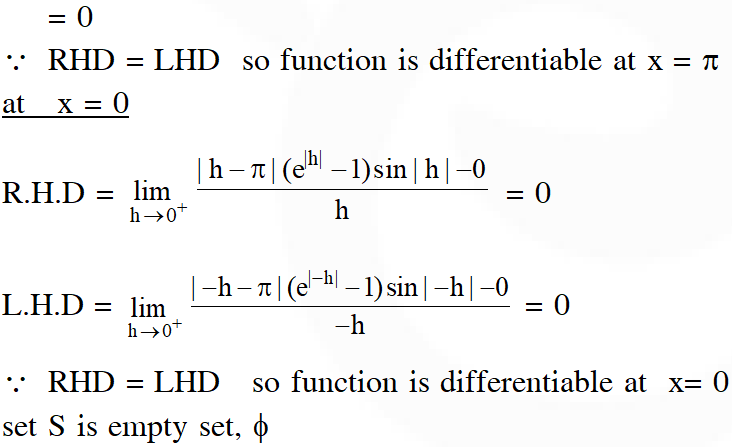Q. Let $\mathrm{S}=\{\lambda, \mu)$ $\varepsilon \operatorname{RxR}: f(t)$ $\left.=(|\lambda|) \mathrm{e}^{|t|}-\mu\right)$. $\sin (2|\mathrm{t}|)$, $\mathrm{t} \varepsilon \mathrm{R},$ is a differentiable function}.Then S is a subset of : (1) $[0, \infty) \times \mathrm{R}$ (2) $\mathrm{R} \times(-\infty, 0)$ (3) $\mathrm{R} \times[0, \infty)$ (4) $(-\infty, 0) \times \mathrm{R}$ [JEE Mains 2018]
Ans. (3)

Dharampal
May 5, 2022, 8:02 p.m.
y=√sin√e^x find dy/dx give me answer
H
June 13, 2021, 6:57 a.m.
These are very less no of questions
sai
Dec. 4, 2020, 10:55 p.m.
happy
sai
Dec. 4, 2020, 10:55 p.m.